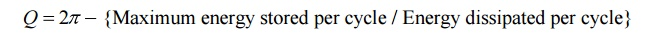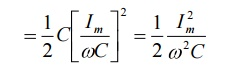Home | | Circuit Theory | Expressions for Q-factor of inductor and capacitor

# Expressions for Q-factor of inductor and capacitor

The resonance phenomenon is observed in ac circuits consisting reactive elements such as inductor and capacitor. These two elements are basic passive elements of energy storing type.

Define Q-factor; Derive the expressions for Q-factor of inductor and capacitor.

(or)

Write a note on figure of merit.

The resonance phenomenon is observed in ac circuits consisting reactive elements such as inductor and capacitor. These two elements are basic passive elements of energy storing type.

It is found convenient to express the efficiency with which these elements store energy. It is also found simpler to compare various inductors and capacitors in terms of efficiency while designing such circuits. Such efficiency is measured as quality factor. It is also called figure of merit.

The figure of merit or Q-factor is defined as= 2pi - {Maximum energy stored per cycle / Energy dissipated per cycle}

Let us derive the expressions for the quality factor of inductor and capacitor.Consider that sinusoidal voltage V is applied to an inductor with leakage resistance R in series as shown in fig.

The maximum energy stored per cycle is given byWhere   Im = Peak or maximum value of current in the circuit.

The average power dissipated in inductor is given by

The total energy dissipated / cycle is given by

Energy dissipated / cycle =Where,        f = frequency of operation.

Thus by definition, the quality factor of inductor is given by,Let us consider that sinusoidal voltage V is applied too capacitor with a small resistance R is series as shown in fig.

The maximum energy stored per cycle is given byWhere Vm = peak or maximum value of applied voltageThe average power dissipated in capacitor is given byThe total energy dissipated per cycle is given by,

Energy dissipated /cycle =where

f = frequency of operation,

Thus by definition, the quality factor of capacitor is given by,Study Material, Lecturing Notes, Assignment, Reference, Wiki description explanation, brief detail
Electrical and electronics : Circuit Theory : Resonance and Coupled Circuits : Expressions for Q-factor of inductor and capacitor |